## Wheatstone Bridge

The Wheatstone bridge circuit is the preferred circuit for measuring resistances. It can be used for the absolute determination of a resistor, or for determining a relative change in resistance. In the measurement with strain gauges, the relative change of resistance is measured.

## Advantages of the bridge circuit

• In a balanced bridge circuit, the output voltage is 0 volts. The gain can be very high in order to achieve a fine resolution.
• The symmetry of the bridge circuit is utilized to compensate for the thermal expansion electrically,
• The symmetry of the bridge circuit is utilized to compensate for unwanted mechanical stretching transversely to the measuring direction electrically.

## Quarter Bridge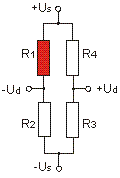Us: bridge supply Ud: bridge output ε: strain k: k-factor (approx. 2,0) Ud/Us = 1/4 · (ΔR1/R1) Ud/Us = 1/4 · k · (ε1) with a K factor k = 2.0 applies:  2000 µm/m corresponding to 1 mV / V

This is the most widely used circuit in the stress analysis.
The active strain gauge (1) is complemented by three passive resistors (2,3,4) for full bridge.

The bridge circuit with only one active strain gauge does not compensate for the temperature-related strain. Therefore, one is dependent on a good self-compensation" of the straingauge: this is achieved by a negative temperature coefficient of the resistance of the strain gauge. This negative temperature coefficient of resistance is set to equal the amount with the change in resistance due to temperature-related strain, but with a negative sign. Therefore, there is strain gauge with "self-compensation" for steel (thermal expansion coefficient 12 ppm/K) and for aluminum (thermal expansion coefficient 23 ppm/K).

The temperature coefficient of resistance is therefore ideally -24 ppm/K at strain gauge for steel and -46 ppm/K for strain gauge for aluminium due to the k-factor with the value 2. The temperature of the resistance (or the indicated strain) as a result of self-compensation is also called apparent strain.

#### Measurement of thermal expansion

The measurement of the thermal expansion of a material is not possible due to the self-compensation of the strain gauge with a quarter bridge. Rather, for this purpose, a second strain gauge R2 of the same properties as R1 is glued to a material sample with the expansion coefficient 0 ppm/K (e.g. Titanium Silicate Glass) and exposed to the same temperature change as R1. It is then a half-bridge. The similar strain gauges are glued to two different materials.

#### Temperature compensation with passive strain gauge in half-bridge circuit

To improve temperature compensation, a second strain gauge R2 is glued to a similar material sample in the stress analysis (with the same thermal expansion as the component to be examined). Both strain gauge R1 and R2 must experience the same temperature change (i.e. installed in the same location). The material sample with R2 (e.g. a steel sheet 25mm x 25mm x 4mm) is elastically (e.g. with silicone) attached to the component.

#### Nonlinearity

The nonlinearity of the quarter bridge is negligible for small strains in the range up to 1000 microns/m.

Rule of thumb: At 1000 microns/m elongation ("at 1 per mille elongation") the deviation of the real elongation from the elongation determined with the linearized bridge equation is about 1

## Half Bridge

#### "Half bridge with two active strain gauges"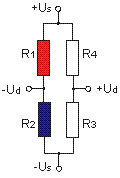Us: bridge supply
Ud: bridge output
ε: strain
k: k-factor (2,0)
Ud / Us = 1/4 · (¨R1 / R1 - ΔR2 / R2)
Ud / Us = 1/4 · k · (ε1 - ε2) with a k-factor k = 2.0 applies:

1000 µm / m corresponding to 1 mV / V

In the (active) half bridge, the two active strain gauge (R1, R2) are supplemented by two passive resistors (R3, R4) to the full bridge. The strain of R1 and R2 must be equal in amount, but opposite sign. For example, R1 is glued to the top, R2 to the bottom of a bending beam.

This circuit is used in low-cost sensors. However, a full bridge can achieve the double output signal.

## Full Bridge

#### "Full bridge with four active strain gauges"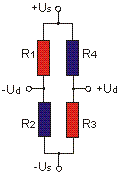Us: bridge supply
Ud: bridge output
ε: strain
k: k-factor (approx. 2,0)
Ud/Us = 1/4 · (ΔR1/R1 - ΔR2/R2 + ΔR3/R3 - ΔR4/R4)
Ud/Us = 1/4 · k · (ε1 - ε2 + ε3 - ε4)

with a k-factor k = 2.0 applies:
500 µm/m correspond to 1 mV/V

The full bridge with 4 active strain gauges is the preferred standard circuit in sensor construction. The strains of R1, R2, R3, and R4 are equal in the amount. The strains of R1 and R3 are each opposite to the strains of R2 and R4.

This circuit provides the largest output signal and the best possible compensation of temperature and mechanical interference.

## Half Bridge

#### Half bridge with two active strain gauges, 1x longitudinal strain, 1x cross strain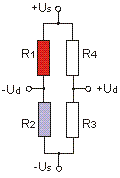Us: bridge supply Ud: bridge output ε: strain k: k-factor (approx. 2,0) ν: Poisson's ratio (approx. 0.3) Ud/Us = 1/4 · (ΔR1/R1 - ΔR2/R2) Ud/Us = 1/4 · k · (ε1 - ν ε2) with a k-factor k = 2.0 applies:  1539 µm/m corresponding to 1 mV/V

The active strain gauge (1) is a transversely disposed "Poisson" strain gauge (2) and two passive resistors (3,4) complements the full bridge.

This circuit is used in the stress analysis and at low cost sensors.

This circuit provides compensation for temperature-related strain provided that the elongation in the direction of the grid of R1 and in the direction of R2 is equal in amount and sign.

In fact, this is not the case with cylindrical bodies: the circumferential elongation is twice as large as the longitudinal elongation. For the relative volume change, the following applies: ΔV/V = 2Δd/d + 1Δl/l due to V=pi d2/4 L

This circuit is rarely used.

## Full Bridge

#### Full bridge with four active strain gauges, 2x longitudinal strains, 2x cross strains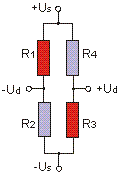Us: bridge supply
Ud: bridge output
ε: strain
k: k-factor (approx. 2,0)
ν: Poisson's ratio (approx. 0.3)
Ud/Us = 1/4 · (ΔR1/R1 - ΔR2/R2 + ΔR3/R3 - ΔR4/R4)
Ud/Us = 1/4 · k · (ε1 - ν ε2 + ε3 - ν ε4)

with a k-factor k = 2.0 applies:
769 µm/m correspond to 1 mV / V

The two co-rotating strain gauge (1.3) are supplemented by two transversely arranged strain gauge (2.4) for the full-bridge.
This circuit is, struts preferably used in tension.
For precision sensors linearization is often provided with additional semiconductor strain gauge.